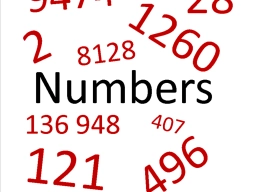# A+B+C=one hundred

A+B+C=100. If A=27.5 and the value of B are between 10 and 15 and which has two decimal places, then what can be the greatest value of C?

C =  62.49

### Step-by-step explanation:Did you find an error or inaccuracy? Feel free to write us. Thank you!